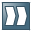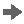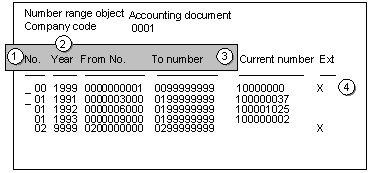Defining Number RangesUse

You can define number ranges as follows:

• You can define number ranges for each company code. Thus, each company code can use the same number interval.
• You can define number range intervals as year-specific.

You define number ranges in the system separately for master records and documents. You can therefore use the same number range keys for both master records and documents.

In the Financial Accounting (FI) component, you can also define alphanumeric number ranges. In this case, however, the document numbers can only be assigned externally.It is advisable to select year-specific number ranges. You therefore only need smaller intervals and can store the document numbers separately according to fiscal years. You therefore avoid documents from the old and new year alternating in January.

Procedure

Since documents may be kept in the system for an indefinite amount of time, you need to define intervals that are large enough to handle this factor.

1. To do this, you need to determine the volume of documents created each year for document types that use the same number range.
1. Multiply the number of documents by the number of years the system can retain a document for. This gives you the required interval capacity.
1. Specify an interval a little larger than that to make sure it is sufficient.
2. In Customizing for Financial Accounting, carry out the activity Define Document Number Ranges under Financial Accounting Global Settings Document Document Number Ranges.
1. For every number range, you must specify the following parameters:
• A key (see 1 in the following diagram)
• A validity date (a year value) until which the number range is valid (see 2 in the following diagram)
• An interval from which the numbers are chosen (see 3 in the following diagram) The intervals for number ranges must not overlap.
• Whether the number range is used in internal or external number assignment (see 4 in the following diagram)